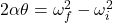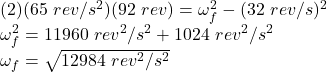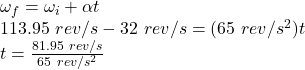Question

A wheel has an angular speed of 32 rev/s when it experiences a constant angular acceleration of 65 rev/s2 which causes it to spin FASTER. During this time the wheel completes 92 rev. Determine how long the wheel was experiencing this angular acceleration and how fast the wheel was spinning at the end of this period. Assume that the wheel doesn’t change the direction of its spin.

1.ωf = 113.95 rev/s

t = 1.26 s

Explanation:

We can use the third equation of motion to find out the final spinning speed of the wheel:where,

α = angular acceleration = 65 rev/s²

θ = No. of revolutions completed = 92 rev

ωf = final angular speed = ?

ωi = initial angular speed = 32 rev/s

Therefore,ωf = 113.95 rev/s

Now, for the time we can use the first equation of motion:t = 1.26 s